FOSHAN TANFON ENERGY TECHNOLOGY CO.,LTDHOME/
What are the main types and parameters of solar inverter?
Published by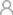FOSHAN TANFON ENERGY TECHNOLOGY CO.,LTD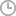December 09,2019

Solar inverter is an important component of the whole solar power generation system. It can directly control the current, voltage and power output of the solar power generation system. In addition, the operation and shutdown of the solar power supply system, as well as the maximum power tracking and restrictions are controlled by the solar inverter, almost all the solar power generator function control, are completed by the inverter.Below, TANFON introduces the main types of solar inverter and its detailed parameters.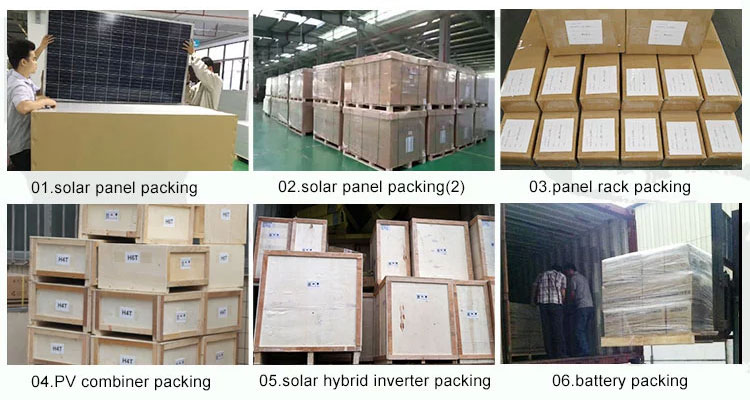According to its use range can be divided into ordinary inverter, charging and inverter all-in-one machine, communications dedicated inverter, aviation military dedicated inverter and so on.In addition, the type of inverter can be divided according to the current waveform output by the inverter.The current output from the inverter can be square wave, ladder wave or sinusoidal wave. For some complete inverters, the current output shape can be adjusted, that is, an inverter can output various types of current.In addition, there are many other ways to divide inverters. For example, according to the frequency of current, they can be divided into low-frequency inverters, medium-frequency inverters and high-frequency inverters, and they can also be divided according to the circuit form of inverters.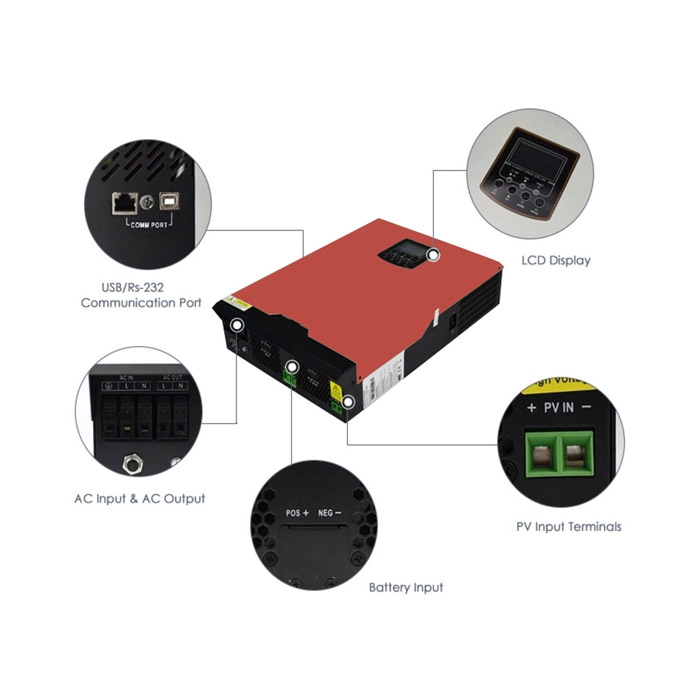There are many main performance parameters of solar inverter, the first consideration should be the use of the inverter environment, solar controller including the use of temperature and altitude, etc..In addition, the rated output voltage of inverter is also the main performance reference index when purchasing.Most solar power generation systems have voltage fluctuations in the output voltage. Some instruments are more precise and require small voltage fluctuations. Most inverters have voltage fluctuations between 3% and 5%.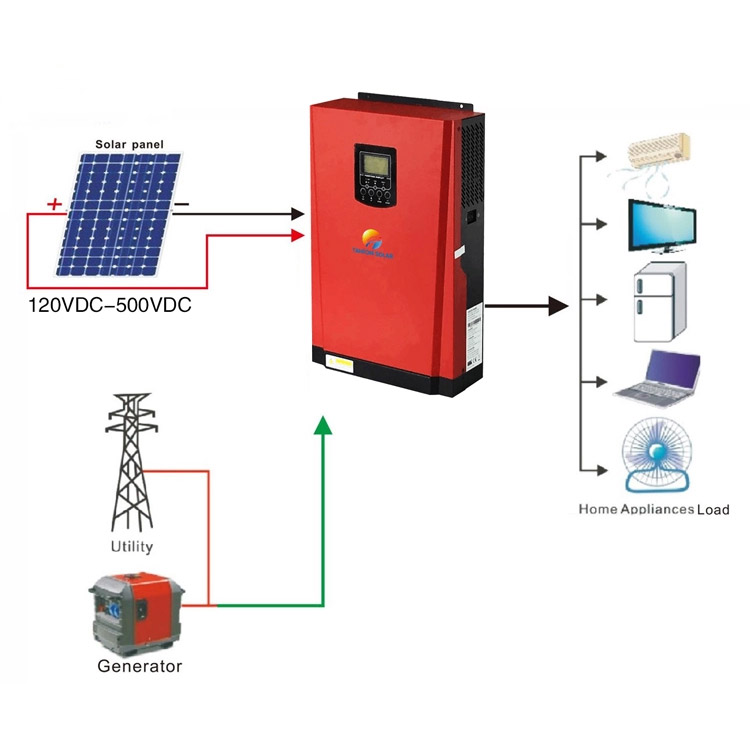###### Do you know how useful a micro inverter is?

Inverter is the core equipment of photovoltaic system. Solar photovoltaic cells convert light energy to dc electricity, which is then converted through the inverter to 50 hz, 230/400 v three-phase or 230v single-phase ac.When using inverters in photovolta

###### Three advantages of solar pv micro inverters

Solar pv micro inverters technology is a kind of MLPE technology, it has been a hot market. According to the data, more than half of the roofs of most photovoltaic power plants use micro-inverters. 1. High safety (1) dc voltage is low In the tr